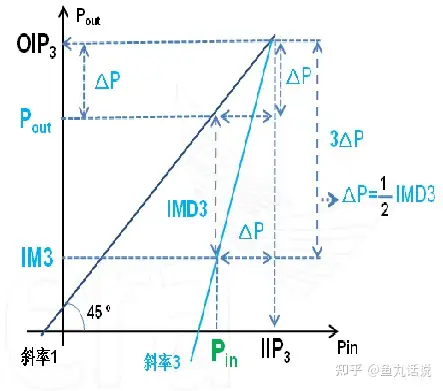# Why is there a difference between the IP3out of ADS simulation OIP3 and the result of self-drawing

#### ianian12377

##### NewbieHi this is the amplifier I am currently designing and want to simulate the OIP3 at POUT 23 dBm,
From this figure, it can be known that when Pin = -13.8 dBm, Gain = 36.8 dB and Pout = 23dBm.Use 2TONE on ADS and set Pin = -13.8 dBm.Using the tool IP3out, the OIP3_lower and OIP3_upper can be obtained as 37.87 dBm and 39.79 dBm respectivelyBut I use the analog method of this picture to draw the picture:It can be known that: IM3_lower = -15.77, IM3_upper = -19.62Draw the intersection points with slopes of 1 and 3, and find that the intersection points will be at 42.39, 44.31

Excuse me, why is there a difference between the junction point of self-drawing and the result of using ADS IP3out? Thank you very much

#### BigBossStart Pin_avs from much lower values and plot the straight lines from this point. You plotted straight lines almost where the nonlinearity starts at.

##Commands Quick-Menu: